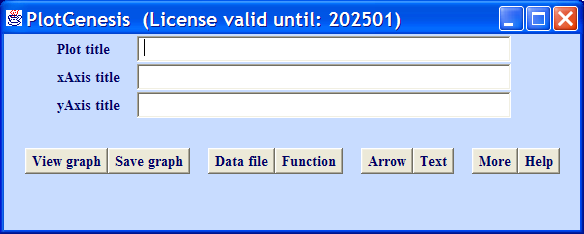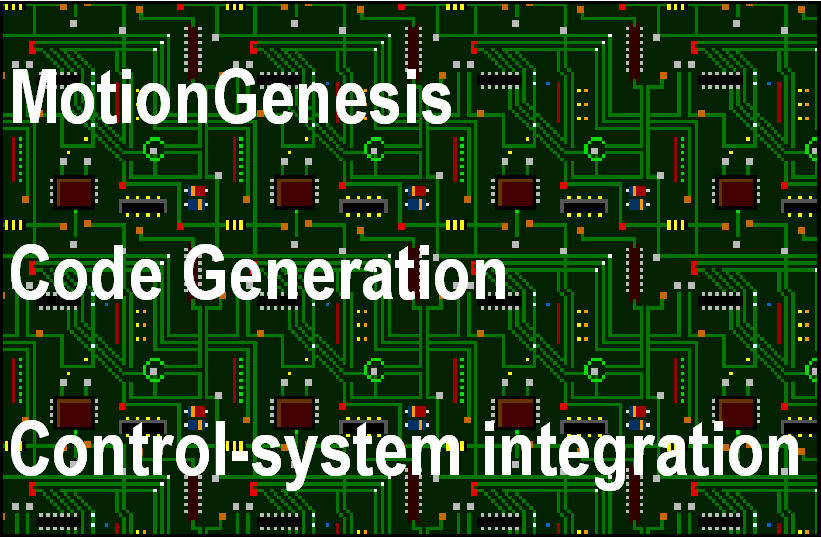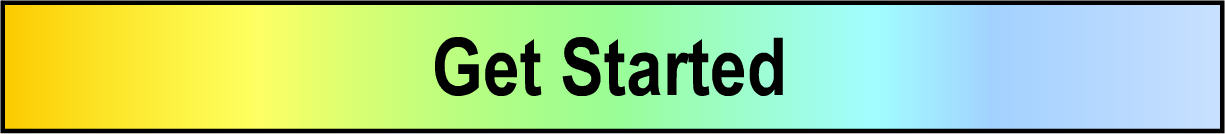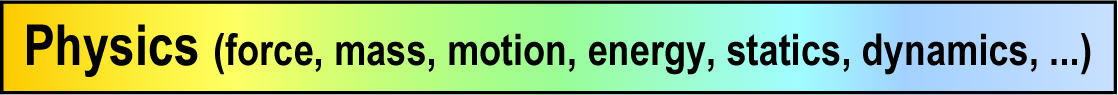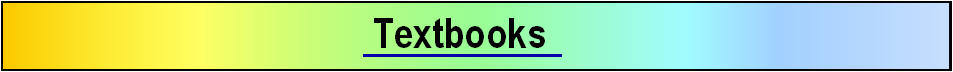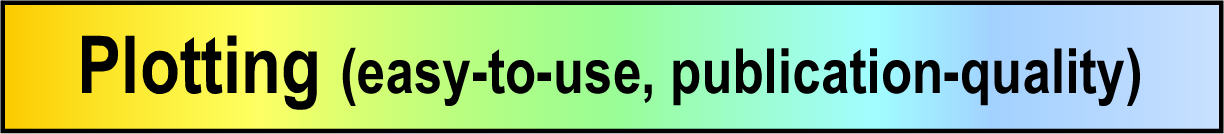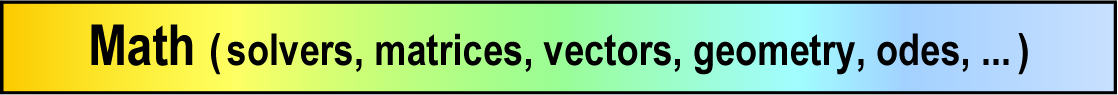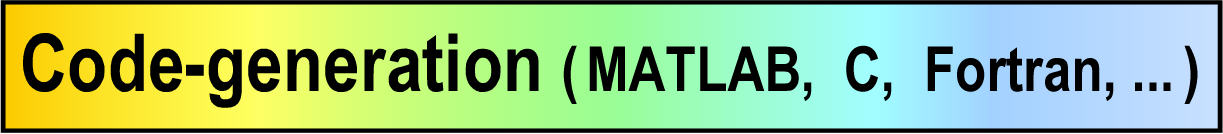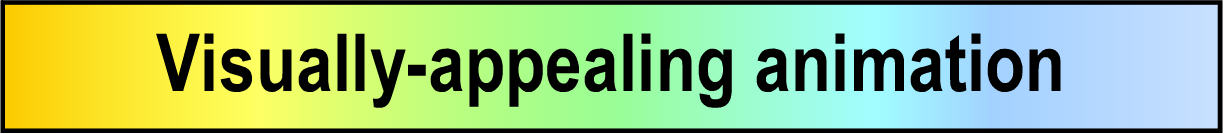MotionGenesis™ Kane is AUTOLEV compatibile
• To run an AUTOLEV file, enter or include the command   SetDefaults( AUTOLEV )
• To be startup AUTOLEV compatible, edit the file MGDefaultPreferences.txt
(in MGToolbox folder) and un-comment  SetDefaults( AUTOLEV )
• With  SetDefaults( AUTOLEV )   runs all AUTOLEV user-manual files with identical output.
• Enhanced MotionGenesis Kane action/reaction law requires the following changes:  Old Command New command Force( Q, Vec> ) Force( P/Q, Vec> ) Q.AddForce( Vec> ) Q.AddForce( P, Vec> ) Torque( B, Vec> ) Torque( A/B, Vec> ) B.AddTorque( Vec> ) B.AddTorque( A, Vec> )Autolev Compatible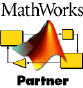New MotionGenesis Kane features for AUTOLEV users
Click  here  for a more complete list of links software features (new and old).

• New code generation:
• Easier to use and modify C, MATLAB®, and Fortran codes.
• Enhanced  units and unit conversions  (also updated with NIST/CODATA 2006 data).
• Improved algorithm for Code Nonlinear with MATLAB® codes.
• SolveSetInput command for solving linear/nonlinear algebraic equations for proper initial values.
• SolveSetInputDt command for enforcing embedded constraints and their time-derivatives.
• New SolveNonlinearSetInput command solves nonlinear algebraic equations and sets initial values
• New symbolic and numeric calculations:
• Enhanced symbolic simplification.
• Built-in solver for differential equations.
• Solve and SolveDt commands solve linear or nonlinear equations.
• Solves linear or nonlinear algebraic equations (recursively), and sets input.
• Evaluate and Solve commands provide unit conversions for their arguments.
• EvaluateToNumber command evaluates expressions at input values.
• ImaginaryNumber names sqrt(-1) for reporting results of complex calculations.
• DtDt command calculates ordinary 2nd derivatives with respect to t.
• SetDt command automates calculation of variable/specified derivatives.
• FactorLinear and FactorQuadratic commands supersede the Arrange command.
• New vectors and geometry:
• Commands for calculating angles and distances.
• Special constructors for vectors and dyadics.
• Creates matrix representations of vectors and dyadics, and vice-versa.
• Calculates unit vectors with GetUnitVector command.
• New statics and dynamics:
• GetStatics command sums forces and moments for static analysis.
• GetStaticsKane command eliminates constraint forces for efficient static analysis.
• GetDynamics command automates Newton/Euler (D'Alembert) dynamics.
• GetDynamicsKane command automates Kane's dynamics and forms efficient equations of motion.
• Points can be associated with rigid objects for "free-body" analysis
• New rotational and translational kinematics:
• Easy-to-use Rotate command sets rotation matrices, angular velocities, angular accelerations.
• Easy-to-use Translate command sets position vectors, velocities, accelerations.
• New commands to set and get angular velocity and angular acceleration.
• New commands to set and get velocity and acceleration.
• New mass, center of mass, inertia, and charge:
• Easy-to-use SetMass, SetInertia, and SetCharge commands.
• Calculates moments/products of inertia, radii of gyration, and other inertia properties.
• Calculates the position, velocity, and acceleration of the mass center of sets of objects.
• New force and torque:
• Easy-to-use GetResultantForce and GetMomentOfForces commands.
• Simple commands to add forces from springs, dampers, electrostatics, gravity, linear actuations, etc.
• New SetCharge and GetCharge commands facilitate electrostatic force calculations.
• New syntax for Newton's law of action/reaction for forces and torques.
• More efficient calculation of generalized forces.
• New kinetic energy, potential energy, power, and work:
• GetKineticEnergy forms kinetic energy for a particle, rigid body, or system.
• Calculates gravitational and spring potential energies.
• GetPower forms system power and facilitates calculating work from dissipative forces.
• Efficient energy integral for checking numerical integration accuracy
• New advanced methods and constraints:
• GeneralizedSpeed declaration for Kane's method.
• Easier-to-use augmented or embedded constraints.
• SolveSetInput command for enforcing constraints with proper initial values.
• SolveSetInputDt command for enforcing embedded constraints and their time-derivatives.
• New physical objects:
• Enhanced keywords RigidBody, RigidFrame, and NewtonianFrame.
• Declaration Point Q(B) creates point Q as a point of RigidBody (or RigidFrame) B.
• "Origin" point Bo and center of mass Bcm automatically created with declaration RigidBody B.
• New defaults:
• Basis vectors Bx>, By>, Bz> are automatically created with RigidBody (or RigidFrame) B.
• New command SetBasisSubscripts for changing names of basis vectors, e.g.,to B1>, B2>, B3>.
• ImaginaryNumber names sqrt(-1) for reporting results of complex calculations.
• SetDefaults( AUTOLEV )   sets defaults to be compatible with AUTOLEV
• Enhanced plotting: Versatile, easy-to-use, visually appealing  plotting software